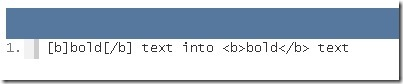# PHP BBCode Parser

BB code is a very simple set of instructions (also known as BB tags) that provide rules as to how a piece of text should be formatted. When a page that contains BB code is displayed in the browser, the BB tags are replaced with appropriate HTML tags that the browser can understand.`<?phpfunction BBCode (\$string) {    \$search = array(        \'\[b\](.*?)\[\/b\]\\',        \'\[i\](.*?)\[\/i\]\\',        \'\[u\](.*?)\[\/u\]\\',        \'\[img\](.*?)\[\/img\]\\',        \'\[url\=(.*?)\](.*?)\[\/url\]\\',        \'\[code\](.*?)\[\/code\]\\'    );    \$replace = array(        \'<b>\\1</b>\',        \'<i>\\1</i>\',        \'<u>\\1</u>\',        \'<img src="\\1">\',        \'<a href="\\1">\\2</a>\',        \'<code>\\1</code>\'    );    return preg_replace(\$search, \$replace, \$string);}?> `
`<?phpecho BBCode(\'Here is some [b]Bold![/b] and so on!\');?>`

Source

http://www.sitepoint.com/bb-code-php-application/

http://www.pixel2life.com/forums/index.php?/topic/10659-php-bbcode-parser/